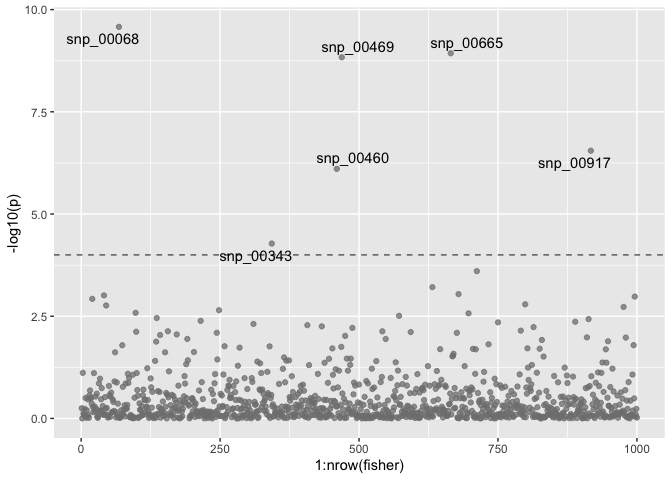# Illustrating multivariate MAPIT with Simulated Data

#### 2023-09-21

If you use multivariate MAPIT in published research, please cite:

# Getting Started

Load necessary libraries. For the sake of getting started, mvMAPIT comes with a small set of simulated data. This data contains random genotype-like data and two simulated quantitative traits with epistatic interactions. To make use of this data, call the genotype data as simulated_data$genotype, and the simulated trait data as simulated_data$trait. The vignette traces the analysis of simulated data. The simulations are described in vignette("simulations").

For a working installation of mvMAPIT please look at theREADME.md or vignette("docker-mvmapit")

library(mvMAPIT)
library(dplyr)
library(ggplot2)
library(ggrepel)
library(tidyr)
library(kableExtra)
library(RcppAlgos)

# Running mvMAPIT

The R routine mvmapit can be run in multiple modes. By default it runs in a hybrid mode, performing tests both wtih a normal Z-test as well as the Davies method. The resulting p-values can be combined using functions provided by mvMAPIT, e.g. fishers_combined(), that work on the pvalues tibble that mvmapit returns.

NOTE: mvMAPIT takes the X matrix as $$p \times n$$; not as $$n \times p$$.

mvmapit_hybrid <- mvmapit(
t(data$genotype), t(data$trait),
test = "hybrid"
)
fisher <- fishers_combined(mvmapit_hybrid$pvalues) To visualize the genome wide p-values, we use a Manhattan plot. The p-values are plotted after combining the results from the multivariate analysis using Fisher’s method. manhplot <- ggplot(fisher, aes(x = 1:nrow(fisher), y = -log10(p))) + geom_hline(yintercept = -log10(thresh), color = "grey40", linetype = "dashed") + geom_point(alpha = 0.75, color = "grey50") + geom_text_repel(aes(label=ifelse(p < thresh, as.character(id), ''))) plot(manhplot)To control the type I error despite multiple testing, we recommend the conservative Bonferroni correction. The significant SNPs returned by the mvMAPIT analysis are shown in the output below. There are in total 6 significant SNPs after multiple test correction. Of the significant SNPs, 4 are true positives. thresh <- 0.05 / (nrow(fisher) / 2) significant_snps <- fisher %>% filter(p < thresh) # Call only marginally significant SNPs truth <- simulated_data$epistatic %>%
ungroup() %>%
mutate(discovered = (name %in% significant_snps$id)) %>% select(name, discovered) %>% unique() significant_snps %>% mutate_if(is.numeric, ~(as.character(signif(., 3)))) %>% mutate(true_pos = id %in% truth$name) %>%
kable(., linesep = "") %>%
kable_material(c("striped"))
id trait p true_pos
snp_00068 fisher 2.65e-10 TRUE
snp_00343 fisher 5.27e-05 FALSE
snp_00460 fisher 7.86e-07 FALSE
snp_00469 fisher 1.47e-09 TRUE
snp_00665 fisher 1.17e-09 TRUE
snp_00917 fisher 2.83e-07 TRUE

## True Epistatic SNPs

To compare this list to the full list of causal epistatic SNPs of the simulations, refer to the following list. There are 5 causal SNPs. Of these 5 causal SNPs, 4 were succesfully discovered by mvMAPIT.

truth %>%
kable(., linesep = "") %>%
kable_material(c("striped"))
name discovered
snp_00068 TRUE
snp_00156 FALSE
snp_00469 TRUE
snp_00665 TRUE
snp_00917 TRUE